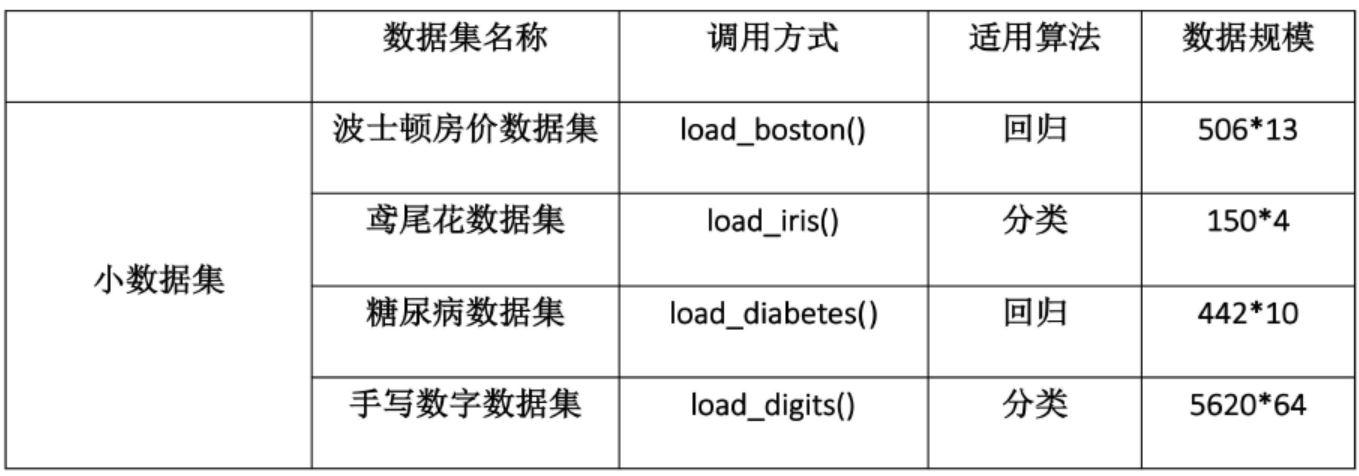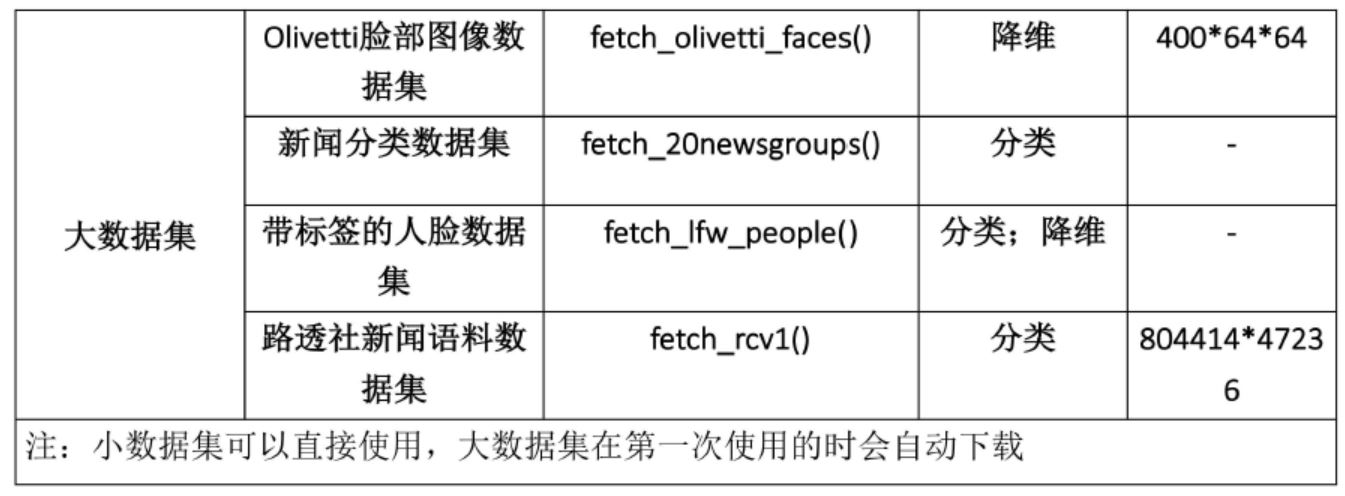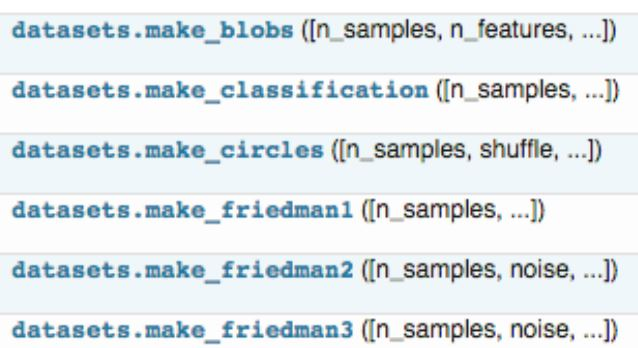# ML神器：sklearn的快速使用及入门

更新时间：2019年07月11日 14:13:40   作者：ML小菜鸟我要评论

1. 获取数据

1.1 导入sklearn数据集

sklearn中包含了大量的优质的数据集，在你学习机器学习的过程中，你可以通过使用这些数据集实现出不同的模型，从而提高你的动手实践能力，同时这个过程也可以加深你对理论知识的理解和把握。（这一步我也亟需加强，一起加油！^-^）

`from sklearn import datasets````iris = datasets.load_iris() # 导入数据集
X = iris.data # 获得其特征向量
y = iris.target # 获得样本label```

1.2 创建数据集```from sklearn.datasets.samples_generator import make_classification

X, y = make_classification(n_samples=6, n_features=5, n_informative=2,
n_redundant=2, n_classes=2, n_clusters_per_class=2, scale=1.0,
random_state=20)

# n_samples：指定样本数
# n_features：指定特征数
# n_classes：指定几分类
# random_state：随机种子，使得随机状可重```
```>>> for x_,y_ in zip(X,y):
print(y_,end=': ')
print(x_)

0: [-0.6600737 -0.0558978  0.82286793 1.1003977 -0.93493796]
1: [ 0.4113583  0.06249216 -0.90760075 -1.41296696 2.059838 ]
1: [ 1.52452016 -0.01867812 0.20900899 1.34422289 -1.61299022]
0: [-1.25725859 0.02347952 -0.28764782 -1.32091378 -0.88549315]
0: [-3.28323172 0.03899168 -0.43251277 -2.86249859 -1.10457948]
1: [ 1.68841011 0.06754955 -1.02805579 -0.83132182 0.93286635]```

2. 数据预处理

`from sklearn import preprocessing`

2.1 数据归一化

```data = [[0, 0], [0, 0], [1, 1], [1, 1]]
# 1. 基于mean和std的标准化
scaler = preprocessing.StandardScaler().fit(train_data)
scaler.transform(train_data)
scaler.transform(test_data)

# 2. 将每个特征值归一化到一个固定范围
scaler = preprocessing.MinMaxScaler(feature_range=(0, 1)).fit(train_data)
scaler.transform(train_data)
scaler.transform(test_data)
#feature_range: 定义归一化范围，注用（）括起来```

2.2 正则化（`normalize`

```>>> X = [[ 1., -1., 2.],
...   [ 2., 0., 0.],
...   [ 0., 1., -1.]]
>>> X_normalized = preprocessing.normalize(X, norm='l2')

>>> X_normalized
array([[ 0.40..., -0.40..., 0.81...],
[ 1. ..., 0. ..., 0. ...],
[ 0. ..., 0.70..., -0.70...]])```

2.3 one-hot编码

one-hot编码是一种对离散特征值的编码方式，在LR模型中常用到，用于给线性模型增加非线性能力。

```data = [[0, 0, 3], [1, 1, 0], [0, 2, 1], [1, 0, 2]]
encoder = preprocessing.OneHotEncoder().fit(data)
enc.transform(data).toarray()```

3. 数据集拆分

```# 作用：将数据集划分为 训练集和测试集
# 格式：train_test_split(*arrays, **options)
from sklearn.mode_selection import train_test_split

X_train, X_test, y_train, y_test = train_test_split(X, y, test_size=0.3, random_state=42)
"""

---
arrays：样本数组，包含特征向量和标签

test_size：
float-获得多大比重的测试样本 （默认：0.25）
int - 获得多少个测试样本

train_size: 同test_size

random_state:
int - 随机种子（种子固定，实验可复现）

shuffle - 是否在分割之前对数据进行洗牌（默认True）

---

(train-test split)
"""```

4. 定义模型

```# 拟合模型
model.fit(X_train, y_train)
# 模型预测
model.predict(X_test)

# 获得这个模型的参数
model.get_params()
# 为模型进行打分
model.score(data_X, data_y) # 线性回归：R square； 分类问题： acc```

4.1 线性回归

```from sklearn.linear_model import LinearRegression
# 定义线性回归模型
model = LinearRegression(fit_intercept=True, normalize=False,
copy_X=True, n_jobs=1)
"""

---
fit_intercept：是否计算截距。False-模型没有截距
normalize： 当fit_intercept设置为False时，该参数将被忽略。 如果为真，则回归前的回归系数X将通过减去平均值并除以l2-范数而归一化。
n_jobs：指定线程数
"""```4.2 逻辑回归LR

```from sklearn.linear_model import LogisticRegression
# 定义逻辑回归模型
model = LogisticRegression(penalty='l2', dual=False, tol=0.0001, C=1.0,
fit_intercept=True, intercept_scaling=1, class_weight=None,
random_state=None, solver='liblinear', max_iter=100, multi_class='ovr',
verbose=0, warm_start=False, n_jobs=1)

"""参数
---
penalty：使用指定正则化项（默认：l2）
dual: n_samples > n_features取False（默认）
C：正则化强度的反，值越小正则化强度越大
n_jobs: 指定线程数
random_state：随机数生成器
fit_intercept: 是否需要常量
"""```

4.3 朴素贝叶斯算法NB

```from sklearn import naive_bayes
model = naive_bayes.GaussianNB() # 高斯贝叶斯
model = naive_bayes.MultinomialNB(alpha=1.0, fit_prior=True, class_prior=None)
model = naive_bayes.BernoulliNB(alpha=1.0, binarize=0.0, fit_prior=True, class_prior=None)
"""

---
alpha：平滑参数
fit_prior：是否要学习类的先验概率；false-使用统一的先验概率
class_prior: 是否指定类的先验概率；若指定则不能根据参数调整
binarize: 二值化的阈值，若为None，则假设输入由二进制向量组成
"""```

4.4 决策树DT

```from sklearn import tree
model = tree.DecisionTreeClassifier(criterion='gini', max_depth=None,
min_samples_split=2, min_samples_leaf=1, min_weight_fraction_leaf=0.0,
max_features=None, random_state=None, max_leaf_nodes=None,
min_impurity_decrease=0.0, min_impurity_split=None,
class_weight=None, presort=False)
"""参数
---
criterion ：特征选择准则gini/entropy
max_depth：树的最大深度，None-尽量下分
min_samples_split：分裂内部节点，所需要的最小样本树
min_samples_leaf：叶子节点所需要的最小样本数
max_features: 寻找最优分割点时的最大特征数
max_leaf_nodes：优先增长到最大叶子节点数
min_impurity_decrease：如果这种分离导致杂质的减少大于或等于这个值，则节点将被拆分。
"""```

4.5 支持向量机SVM

```from sklearn.svm import SVC
model = SVC(C=1.0, kernel='rbf', gamma='auto')
"""参数
---
C：误差项的惩罚参数C
gamma: 核相关系数。浮点数，If gamma is ‘auto' then 1/n_features will be used instead.
"""```

4.6 k近邻算法KNN

```from sklearn import neighbors
#定义kNN分类模型
model = neighbors.KNeighborsClassifier(n_neighbors=5, n_jobs=1) # 分类
model = neighbors.KNeighborsRegressor(n_neighbors=5, n_jobs=1) # 回归
"""参数
---
n_neighbors： 使用邻居的数目
n_jobs：并行任务数
"""```

4.7 多层感知机（神经网络）

```from sklearn.neural_network import MLPClassifier
# 定义多层感知机分类算法
"""参数
---
hidden_layer_sizes: 元祖
activation：激活函数
alpha：L2惩罚(正则化项)参数。
"""```

5. 模型评估与选择篇

5.1 交叉验证

```from sklearn.model_selection import cross_val_score
cross_val_score(model, X, y=None, scoring=None, cv=None, n_jobs=1)
"""参数
---
model：拟合数据的模型
cv ： k-fold
scoring: 打分参数-‘accuracy'、‘f1'、‘precision'、‘recall' 、‘roc_auc'、'neg_log_loss'等等
"""```

5.2 检验曲线

```from sklearn.model_selection import validation_curve
train_score, test_score = validation_curve(model, X, y, param_name, param_range, cv=None, scoring=None, n_jobs=1)
"""参数
---
model:用于fit和predict的对象
X, y: 训练集的特征和标签
param_name：将被改变的参数的名字
param_range： 参数的改变范围
cv：k-fold

---
train_score: 训练集得分（array）
test_score: 验证集得分（array）
"""```

6. 保存模型

6.1 保存为pickle文件

```import pickle

# 保存模型
with open('model.pickle', 'wb') as f:
pickle.dump(model, f)

# 读取模型
with open('model.pickle', 'rb') as f:
model.predict(X_test)```

6.2 sklearn自带方法

```from sklearn.externals import joblib

# 保存模型
joblib.dump(model, 'model.pickle')

#载入模型